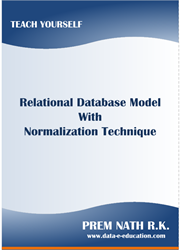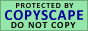Functional Dependency

William Ward Armstrong is a Canadian Mathematician and Computer Scientist. Armstrong invented Axioms of Dependency Concept is known Armstrong's Axioms in the Mathematical Science. Armstrong's Axioms Rules are helps to find out Functional Dependency of Relation that implemented in the Relational Database Model.

Functional Dependency is a constraint between two sets of attributes in a Relation that mathematical concept is helps to design and optimize a Relational Database Structure. Dependency can be categories into two types are "Single Valued Dependency" and "Multivalued Dependency".

Functional Dependencies were first defined by E.F. Codd in the Relational Database Theory based on Single Valued Dependency and then within the last few years Ronald Fagin and independently Zaniolo defined a generalization of Functional Dependencies is called Multivalued Dependencies.

Let r be a tuple of a relation R and A be an attribute of R. Then r(A) is the A is component of r (i.e., the value of r for the attribute A). When, a relation R is defined with set of attributes (A, B, C, D) that can determine È (union). Let X be a subset of È, and let r be a tuple of R. The projection of r onto X, written r[X] is a mapping from the attributes of X to their domains such that r[X](A) = r(A) for all A in X. The projection of the relation R onto X is obtained by projecting each tuple in R onto X and eliminating duplicate tuples.

Functional Dependency is a statement of the form X ® Y, where both X and Y are sets of attributes. A relation R satisfies the functional dependency X ® Y (or X ® Y holds in R) if for every pair r1, r2 of tuples of R, if r1[X] = r2[X], then r1[Y] = r2[Y].

An instance, a relation R, a set of attributes X in R is said to functionally determine another attribute Y in R, IF and Only IF each X value is related with exactly one Y value is called Functional Dependency. IFF (IF and Only IF) is a biconditional logical connective between statements based on combination of material conditional (IF) and Reverse (Only IF) statements.

In the relation R, attribute X is a determinant set and attribute Y is a dependent attribute. Each value of the attributes in X can determine with corresponding value of the Y attribute. X ® Y symbolize that X functionally determines each of dependent set members of Y.

Dependency Rules

Inference Rules are helps to determine when a dependency is implied by some other dependencies. An instance, a dependency s is a consequence of a set of dependencies S if for all relation R, s holds in R if all the dependencies of S hold in R. These rules are sound and complete in the sense that s is a consequence of S if and only if s can be inferred from S by a sequence of applications of the rules.

The rules are divided into three groups that reflecting the fact that the set S È {s} may hold only functional dependencies or only multivalued dependencies, or a combination of functional and multivalued dependencies.Your Comment To AUTHORDownload e-Book